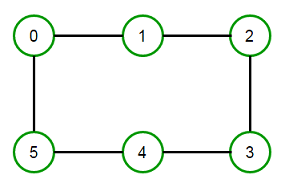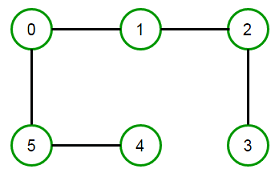# Determine if an undirected graph is a Tree (Acyclic Connected Graph)

Given an undirected graph, check if is is a tree or not. In other words, check if given undirected graph is a Acyclic Connected Graph or not.

For example, the graph shown on the right is a tree and the graph on the left is not a tree as it contains a cycle 0-1-2-3-4-5-0.A tree is an undirected graph in which any two vertices are connected by exactly one path. In other words, any acyclic connected graph is a tree. We can easily determine acyclic connected graph by doing DFS traversal on the graph. When we do a DFS from any vertex v in an undirected graph, we may encounter back-edge that points to one of the ancestors of current vertex v in the DFS tree. Each “back edge” defines a cycle in an undirected graph. If the back edge is `x -> y`, then since y is ancestor of node x, we have a path from y to x. So we can say that we have a path `y ~~ x ~ y` that forms a cycle. (Here `~~` represents one more more edges in the path and `~` represents a direct edge) and is not a tree.

## C++

Output:

Graph is not a Tree

## Java

Output:

Graph is not a Tree

The time complexity of above solution is O(n + m) where n is number of vertices and m is number of edges in the graph.(1 votes, average: 5.00 out of 5)Loading...